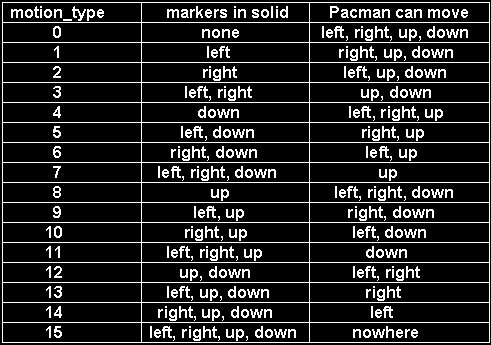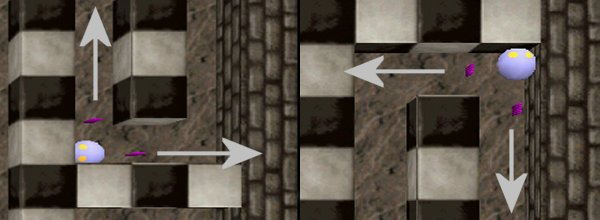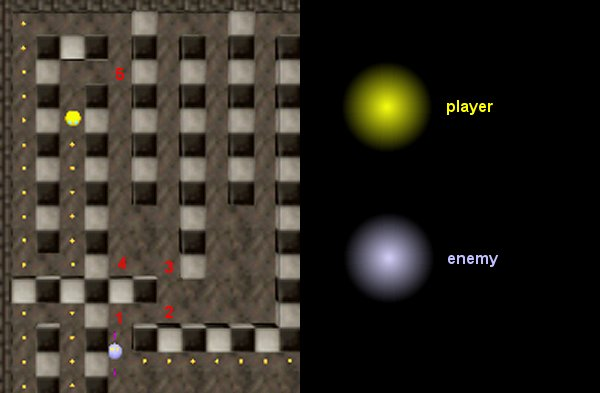Code snippets
 Pacmen I remember myself playing Pacman on a Sinclair Spectrum computer. These were the days! If you were a programmer, you had to make sure that your game has less than 48kb - or it wouldn't fit in the memory! Pacmen uses a "little" more memory - it is a Pacmen (several men :) not Pacman. Function main has nothing special in it; let's take a look at the action that drives our hero: action pacman {     player = me;     my.enable_entity = on;     my.enable_push = on;     my.enable_impact = on;     my.event = kill_me; // here's what happens on collision with enemies     camera.diameter = 0; // camera can pass through walls     camera.tilt = -90; // looks down     camera.z = my.z + 1000; // 1000 quants above the player     pacman_speed.y = 0;     pacman_speed.z = 0; Pacman is sensitive to other entities; if it collides / is run over by enemies, its kill_me event is triggered. The camera can pass through walls, looks down, moves with the player and is placed 1000 quants above the player. Pacman and its enemy are using invisible "antennas" (markers) to detect their environment and act like smart creatures. I have used this technique in my first AI demo in AUM. In this picture you can see the pink antennas (run the game with pacmen -d markers if you want to see them too):We are using four markers for pacman and four markers for the enemy, like below:Let's see what's with these antennas; I'll explain what's happening with the left marker (the code for the other markers is the same):    while (player != null)    {          motion_type = 0; As long as the player is alive (player != null) we reset motion_type (more on that later). We are using rotated coords for all the markers because the player and enemy are rotated models, too.          player_left.x = my.x;          player_left.y = my.y + width;          player_left.z = my.z;          result = content(player_left);          if (result == content_solid) {motion_type += 1;} ............................................................................................... We check the content of the player_left marker and if it is in solid, we add 1 to motion_type. If player_right == content_solid -> motion_type += 2, player_down == content_solid -> motion_type += 4, player_up == content_solid -> motion_type += 8. I chose these binary values (1, 2, 4, 8) to make sure that they represent unique combinations of numbers. If the markers for right and up are in solid, motion_type will be 2 + 8 = 10 and this is the only combination who's result will be equal to 10. Look at the table below to see all the combinations:It is clear that motion_type = 15 can never happen, but I wanted to show you all the possibilities. So we have four markers, we check their content and depending on the result, we allow them (pacman and enemy) to move only if they choose a direction where the road is "clear".   if (motion_type == 0)   {       if (key_cuu == 1) {my.pan = 0;}       if (key_cud == 1) {my.pan = 180;}       if (key_cur == 1) {my.pan = 270;}       if (key_cul == 1) {my.pan = 90;} } if (motion_type == 1) {       if (key_cuu == 1) {my.pan = 0;}       if (key_cud == 1) {my.pan = 180;}       if (key_cur == 1) {my.pan = 270;} } if (motion_type == 2) {       if (key_cuu == 1) {my.pan = 0;}       if (key_cud == 1) {my.pan = 180;}       if (key_cul == 1) {my.pan = 90;} } if (motion_type == 3) {       if (key_cuu == 1) {my.pan = 0;}       if (key_cud == 1) {my.pan = 180;} } if (motion_type == 4) {       if (key_cuu == 1) {my.pan = 0;}       if (key_cur == 1) {my.pan = 270;}       if (key_cul == 1) {my.pan = 90;} } if (motion_type == 5) {       if (key_cuu == 1) {my.pan = 0;}       if (key_cur == 1) {my.pan = 270;} } if (motion_type == 6) {       if (key_cuu == 1) {my.pan = 0;}       if (key_cul == 1) {my.pan = 90;} } if (motion_type == 7) {       if (key_cuu == 1) {my.pan = 0;} } if (motion_type == 8) {       if (key_cud == 1) {my.pan = 180;}       if (key_cur == 1) {my.pan = 270;}       if (key_cul == 1) {my.pan = 90;} } if (motion_type == 9) {       if (key_cud == 1) {my.pan = 180;}       if (key_cur == 1) {my.pan = 270;} } if (motion_type == 10) {       if (key_cud == 1) {my.pan = 180;}       if (key_cul == 1) {my.pan = 90;} } if (motion_type == 11) {       if (key_cud == 1) {my.pan = 180;} } if (motion_type == 12) {       if (key_cur == 1) {my.pan = 270;}       if (key_cul == 1) {my.pan = 90;} } if (motion_type == 13) {       if (key_cur == 1) {my.pan = 270;} } if (motion_type == 14) {       if (key_cul == 1) {my.pan = 90;} } Now if the following line won't give you a headache then nothing else will: if ((my.pan == 0 && motion_type < 8) || ((my.pan == 90) && (motion_type % 2 != 1)) || (my.pan == 180 && motion_type != 4 && motion_type != 5 && motion_type != 6 && motion_type != 7 && motion_type != 12 && motion_type != 13 && motion_type != 14) || (my.pan == 270 && motion_type != 2 && motion_type != 3 && motion_type != 6 && motion_type != 7 && motion_type != 10 && motion_type != 11 && motion_type != 14)) {   ent_move (pacman_speed, nullvector); } Well, this line is pretty simple: it stops the movement when the markers are in solid because we don't want Pacman to stick its nose into the wall, isn't it? If Pacman is pointing its "nose" in the right direction (the road is clear) ent_move is executed so Pacman moves. I have used several barbarian techniques in this instruction: motion_type % 2 != 1 replaces motion_type != 1 && motion_type != 3 .... && motion_type != 13 (all the odd numbers between 1 and 13). The action attached to the enemy is similar; the only big difference is that we generate a random number and depending on its value we set the direction for the enemy.If you look at the first example, the enemy is coming from right to the left; the available ("free") directions are up and right. The enemy came from the right so it wouldn't look that clever if it would bounce off the wall and return where it came from so this possibility has been disabled in enemy's code. In this case, the enemy will go up. In the second example, the enemy is coming going up and it won't return down, but move to the left because I set this limit in its code for this situation. The enemy can pass through dots so I am using this line in its action (the dots are passable): move_mode = ignore_passents; Here's the action attached to every dot: action dot {     my.push = -1;     my.z = player.z;     my.passable = on;     while (my != null && player != null)     {         if (vec_dist (my.x, player.x) < 30)         {             play_sound (eatdot_snd, 100);             ent_remove (my);             score += 10;        }        wait (1);    } } The dots are aligned to player's z; this way I don't need to make sure that I place them at the proper height in Wed. If the player comes closer than 30 quants, the dot will be eaten and the score will be increased by 10. The function that kills the player is simple; player's scale is lowered 10 times and then the player is removed. function kill_me() {     exclusive_global;     play_sound gotme_snd, 100;     while (my.scale_x > 0.1)     {         my.scale_x -= 0.1 * time;         my.scale_y = my.scale_x;         my.pan += 1 / my.scale_x;         wait (1);     }     waitt (2);     ent_remove (me); } If you want to move to the next level, check the score in a loop; score = 600 can take you to the next level if you have 60 dots in the level. What about "real" AI? This enemy of yours does nothing but moves correctly in the level! This part is easy and is left as an exercise for you. Pacmen has four different enemies - I've coded the dumb one. Don't worry - I'll explain how to code the most Pac-thirsty enemy right here:The enemy must make sure that its x and y coordinates are equal with player's x, y coords (this means that the enemy has "got" the player). When the enemy reaches the 1st red point, it can't come closer to the player - it must move to the right, towards the 2nd point. When it arrives there its motion type has changed; the enemy knows that by moving upwards the distance between it and the player is getting smaller (on y) so it will move towards the 3rd point (we aren't using any random value here). By choosing to move towards the 4th point (again, not a "random" decision) the enemy knows that the distance between it and the player (on x) will get smaller. The 5th point can be a random decision or not, depending on player's position. And you thought that creating a Pacman clone is easy!   Shooting switches / statues This piece of code will show you how you can move to the next level only if you have destroyed a certain number of idols / statues / switches / whatever you want. action statue {    my.enable_shoot = on;    my.event = destroy_me;    statues_left += 1;    while (1)    {        if (vec_dist(my.x, player.x) < 50 || my.skill10 == 1)        {            play_sound (destroyed_snd, 70);            statues_left -= 1;            _gib (10);            ent_remove (me);            return;       }       wait (1);    } } Every statue is sensitive at shooting; their number is counted at startup. If the player comes close to a statue (or shoots it) a sound is played, the number of statues is decreased, the statue breaks in pieces and disappears. What about shooting? Here's the event that is triggered when the statue is shot: function destroy_me() {     if (event_type == event_shoot)     {          my.skill10 = 1;     }     else     {          my.skill10 = 0;     } } It doesn't look like having something to do with the statues, isn't it? If you look at action statue above you'll see that the statue is destroyed if the player comes close to it OR if skill10 = 1 - that's what the event does. We must have another entity that takes us to the next level on impact: action next_level {    my.enable_impact = on;    my.event = change_level; } When we impact with this entity, its change_level event will be triggered: function change_level() {        if (statues_left != 0) {return;}        me = null;        beep; beep;        // load_level ;         wait (2); } You can see that we can't move to the next level if we haven't destroyed all the statues. You will have to remove the comment for the load_level line and change the name to your_next_level.wmb